# MOTION IN A STRAIGHT LINE

### BASIC DEFINITIONS

Mechanics : Branch of physics, which deals with the study of objects in rest and in motion.
Statics : Study of objects at rest or in equilibrium.

Kinematics : Study of motion of objects without considering the cause of motion.

Dynamics : Study of motion of objects considering the cause of motion.

Rest  : An object is said to be at rest if it does not change its position with time, with respect to its surrounding (a reference point which is generally taken as origin in numerical problems)

Motion : An object is said to be in motion if it changes its position with time, with respect to its surroundings.
Rest and motion are relative terms.

Point mass/Point object : An object is said to be a point mass if during its motion it covers distance much greater than its own size.

One dimensional motion : An object travels in a straight line. It is also called rectilinear or linear motion. The position change of the object with time in one dimension can be described by only one coordinate.
Ex. A stone falling freely under gravity.

Two dimensional motion or motion in a plane : For an object travelling in a plane two coordinates say X and Y are required to describe its motion.
Ex. An insect crawling over the floor.

Three dimensional motion : An object travels in space. To describe motion of objects in three dimension require all three coordinates x, y and z.
Ex. A kite flying in the sky.

### DISTANCE AND DISPLACEMENT

Distance or Path length : The length of the actual path travelled by an object during motion in a given interval of time is called the distance travelled by that object or path length. It is a scalar quantity.

Displacement : It is the shortest distance between the initial and final position of an object and is directed from the initial position to the final position. It is a vector quantity.

KEEP IN MEMORY
1. Displacement may be positive, negative or zero but distance is always positive.
2. Displacement is not affected by the shift of the coordinate axes.
3. Displacement of an object is independent of the path followed by the object but distance depends upon path.
4. Displacement and distance both have same unit as that of length i.e. metre.
5.6. For a moving body distance always increases with time
7. For a body undergoing one dimensional motion, in the same direction distance = | displacement |. For all other motion distance > | displacement |.

### SPEED

It is the distance travelled per unit time by an object. It is a scalar quantity. It cannot be negative.

Uniform speed : An object is said to be moving with a uniform speed, if it covers equal distances in equal intervals of time, howsoever small the time intervals may be.

Non-uniform speed : If an object covers unequal distances in equal interval of time or equal distances in unequal interval of time.

Instantaneous speed : The speed of an object at a particular instant of time is called the instantaneous speed.
Vinst =Average speed : It is ratio of the total distance travelled by the object to the total time taken.Dimensions : [M0LT-1]
Unit : In SI systems.

### VELOCITY

It is the displacement of an object per unit time. It is a vector quantity. It can be positive negative or zero.

Uniform velocity :  An object is said to be moving with a uniform velocity, if it covers equal displacements in equal intervals of time, howsoever small the time intervals may be.

Non-uniform velocity : If an object covers unequal displacements in equal interval of time or equal displacements in unequal interval of time.

Instantaneous velocity : The velocity of an object at a particular instant of time is called the instantaneous velocity.Average Velocity : It is ratio of the total displacement to the total time taken.Dimensions : [M0LT–1]
Unit : In SI system, m/s

KEEP IN MEMORY
1. | Average velocity | can be zero but average speed cannot be zero for a moving object.
2.3. | Instantaneous velocity | = Instantaneous speed.
4. A particle may have constant speed but variable velocity. It happens when particle travels in curvilinear path.
5. If the body covers first half distance with speed v1 and next half with speed v2 then
Average speed1. If a body covers first one-third distance at a speed v1, next one-third at speed v2 and last one-third at speed v3, then
Average speed1. If a body travels with uniform speed v1 for time t1 and with uniform speed v2 for time t2, then
Average speed### ACCELERATION

The rate of change of velocity with respect to time is called acceleration. It is a vector quantity.
Let velocity changes byduring some interval of time.
Average accelerationis given byInstantaneous accelerationis given bySI unit is meter/sec2 (ms–2).
A body moving with uniform velocity has zero acceleration. It means that neither its speed nor its direction of motion is changing with time.
Uniform acceleration : If the velocity of the body changes in equal amount during same time interval, then the acceleration of the body is said to be uniform.  Acceleration is uniform when neither its direction nor magnitude changes with respect to time.

Variable or non-uniform acceleration : If the velocity of body changes in different amounts during same time interval, then the acceleration of the body is known as variable acceleration. Acceleration is variable if either its direction or magnitude or both changes with respect to time. A good example of variable acceleration is the acceleration in uniform circular motion.

### EQUATIONS FOR UNIFORMLY ACCELERATED MOTION

When the motion is uniformly accelerated i.e., when acceleration is constant in magnitude and direction :
• v = u + at
•• v2 – u2 = 2as
where u = initial velocity
v = final velocity
a = uniform acceleration
s = distance travelled in time t
•• Distance travelled in nth second
sn =;
sn = distance covered in nth second

Above equations in vector formWhen displacement (s) is given as a function of time t [s = f(t)] then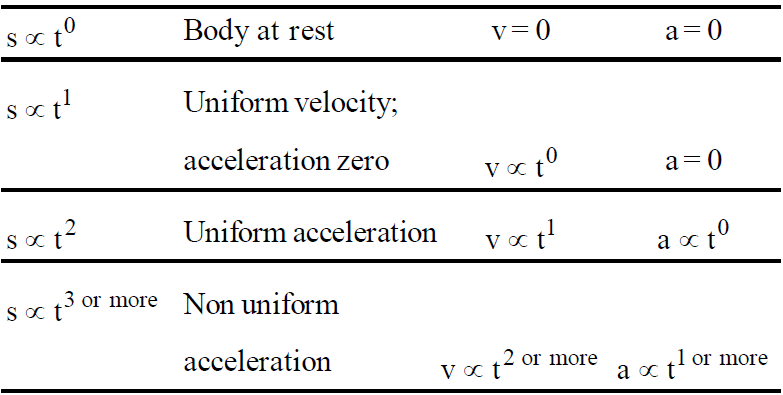We use calculus method (integration and differentiation) for displacement, velocity, acceleration as a function of time.
We know that;;
when a = f(s),
where s = displacement, v = instantaneous velocity, a = instantaneous acceleration

### VERTICAL MOTION UNDER GRAVITY

• For a body thrown downward with initial velocity u from a height h, the equations of motion are
v = u +gt• If initial velocity is zero, then the equations are
v = gt• When a body is thrown upwards with initial velocity u, the equations of motion are
v = u – gtv2 = u2   2gh
To summarise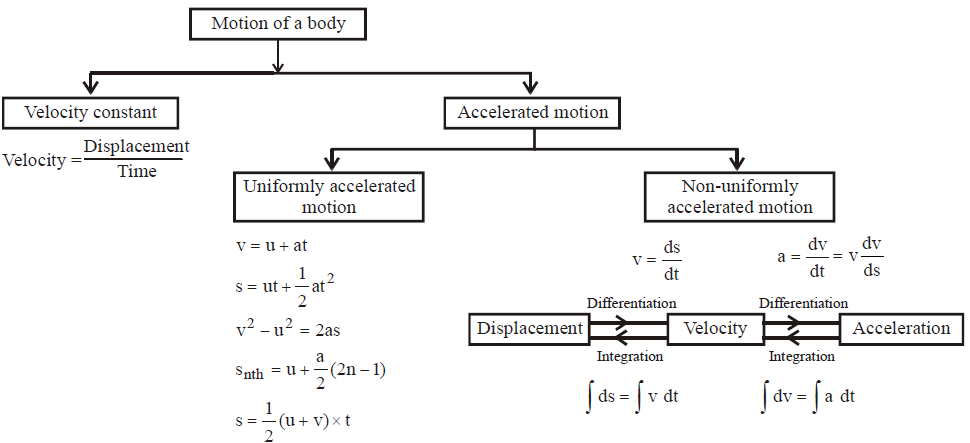Note:- Calculus method as shown in non-uniformly accelerated motion may also be used for uniformly accelerated motion.

### UNIFORMLY ACCELERATED MOTION : A DISCUSSION

While using equations of motion we can have two approaches.
Approach 1 : Take a = +ve when velocity increases and a = –ve when velocity decreases.
Take rest of physical quantities such as u, v, t and s as positive.

Approach 2 : (Vector method)
Assume one direction to be positive and other negative. Assign sign to all the vectors (u, v, a, s), +ve sign is given to a vector which is directed to the positive direction and vice-versa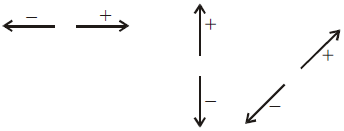Normally the direction taken is as drawn above. But it is important to note that you can take any direction of your choice to be positive and the opposite direction to be negative.

Note:-  The second method (or approach) is useful only when there is reversal of motion during the activity concerned.

KEEP IN MEMORY
1. The direction of average acceleration vector is the direction of the change in velocity vectorhas a direction ofi.e., the resultant ofand1. There is no definite relationship between velocity vector and acceleration vector.
2. For a body starting from rest and moving with uniform acceleration, the ratio of distances covered in t1 sec.,
t2 sec, t3 sec, etc. are in the ratio t12 : t22 : t32 etc.
3. A body moving with a velocity v is stopped by application of brakes after covering a distance s. If the same body moves with a velocity nv, it stops after covering a distance n2s by the application of same retardation.

KEEP IN MEMORY
1. An object moving under the influence of earth's gravity in which air resistance and small changes in g are neglected is called a freely falling body.
2. In the absence of air resistance, the velocity of projection is equal to the velocity with which the body strikes the ground.
3. Distance travelled by a freely falling body in 1st second is always half of the numerical value of g or 4.9 m, irrespective of height h.
4. For a freely falling body with initial velocity zero
1. Velocity ∝ time (v = gt)
2. Velocity(v2 = 2gs)
3. Distance fallen α (time)2, where g is the acceleration due to gravity.
5. If maximum height attained by a body projected vertically upwards is equal to the magnitude of velocity of projection, then velocity of projection is 2g ms–1 and time of flight is 4 sec.
6. If maximum height attained by a body projected upward is equal to magnitude of acceleration due to gravity i.e., 'g', the time of ascent issec. and velocity of projection is.
7. Ratio of maximum heights reached by different bodies projected with velocities u1, u2, u3 etc. are in the ratio ofetc. and ratio of times of ascent are in ratio of u1 : u2 : u3 etc.
8. During free fall velocity increases by equal amount every descend and distance covered during 1st, 2nd, 3rd seconds of fall, are 4.9m, 14.7m, 24.5m.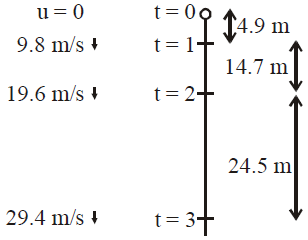1. If a body is projected horizontally from top of a tower, the time taken by it to reach the ground does not depend on the velocity of projection, but depends on the height of tower and is equal to.
2. If velocity v of a body changes its direction by θ without change in magnitude then the change in velocity will be.
3. From the top of a tower a body is projected upward with a certain speed, 2nd body is thrown downward with same speed and 3rd is let to fall freely from same point thenwhere t1 = time taken by the body projected upward,
t2 = time taken by the body thrown downward and
t3 = time taken by the body falling freely.
1. If a body falls freely from a height h on a sandy surface and it buries into sand upto a depth of x, then the retardation produced by sand is given by.
2. In case of air resistance, the time of ascent is less than time of descent of a body projected vertically upward i.e. ta < td.
3. When atmosphere is effective, then buoyancy force always acts in upward direction whether body is moving in upward or downward direction and it depends on volume of the body. The viscous drag force acts against the motion.
4. If bodies have same volume but different densities, the buoyant force remains the same.

CAUTION : Please note that dropping body gets the velocity of the object but if the object is in acceleration, the body dropped will not acquire the acceleration of the object.

COMMON DEFAULT

Incorrect. In the question, if it is given that a body is dropped, taking its initial velocity zero.
Correct. The initial velocity is zero if the object dropping the body is also at rest (zero velocity). But if the object dropping the body is having a velocity, then the body being dropped will also have initial velocity which will be  same as that of the object.
For example :
• When an aeroplane flying horizontally drops a bomb.
• An ascending helicopter dropping a food packet.
• A stone dropped from a moving train etc.

Incorrect. Applying equations of motion in case of non-uniform acceleration of the body.
Correct. The equations of motion are for uniformly accelerated motion of the body.
Please note that when the case is of non-uniform acceleration we use calculus (differentiation and integration).In fact calculus method is a universal method which can be used both in case of uniform as well as non-uniform acceleration.

Incorrect. Taking average velocity same as that of instantaneous velocity.
Correct. Average velocity(whereis position vector at time ti andis position vector at time  tf).
Whereas instantaneous velocityIt is important to note that average velocity is equal to instantaneous velocity only when the case is of  uniform velocity.

Incorrect. Taking acceleration as negative (– a) even when acceleration is an unknown.
Correct. Take acceleration as (a) when it is unknown even if we know that the motion is a case of deceleration or retardation. On solving, we will find the value of (a) to be negative .

Incorrect. Magnitude of instantaneous velocity is different from instantaneous speed .
Correct. Magnitude of instantaneous velocity is equal to the instantaneous speed in any case.

### VARIOUS GRAPHS RELATED TO MOTION

#### DISPLACEMENT-TIME GRAPH

In this graph time is plotted on x-axis and displacement on y-axis.
• For a stationary body (v = 0) the time-displacement graph is a straight line parallel to time axis.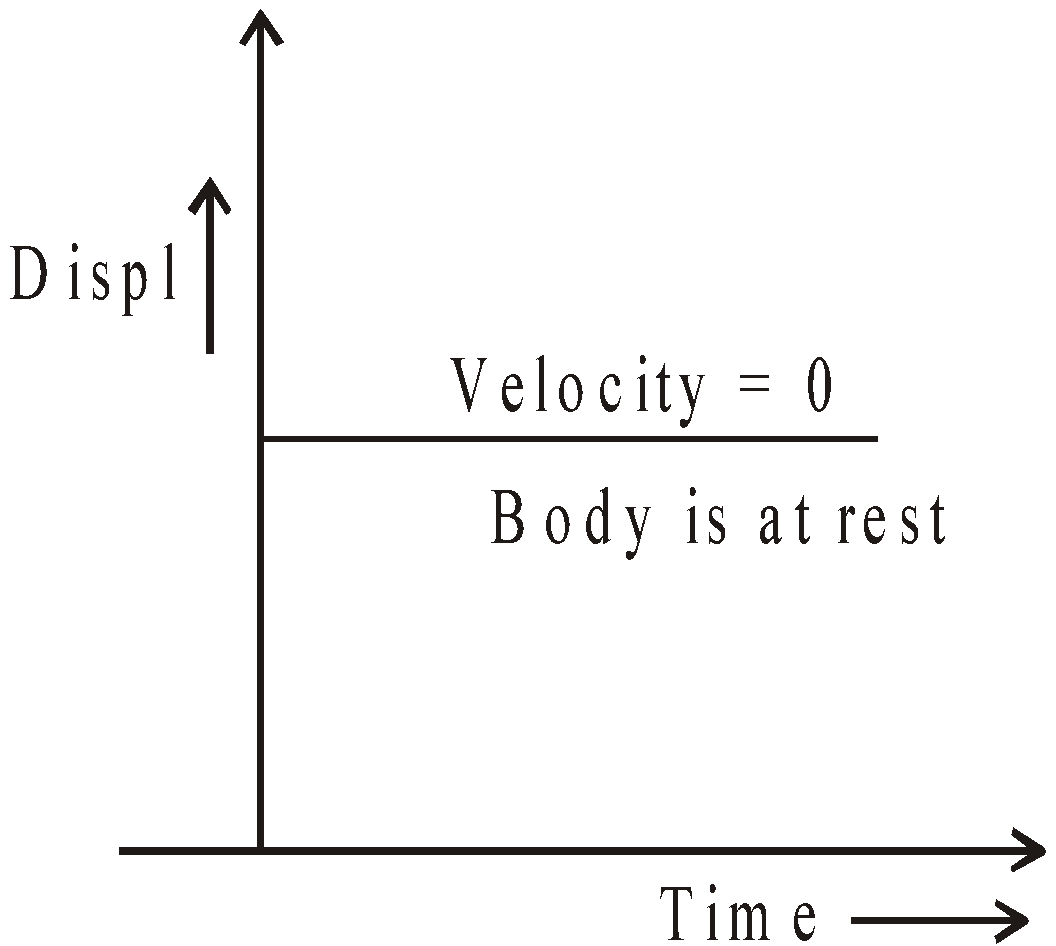• When the velocity of a body is constant then time-displacement graph will be an oblique straight line. Greater the slopeof the straight line, higher will be the velocity.• If the velocity of a body is not constant then the time-displacement curve is a zig-zag curve.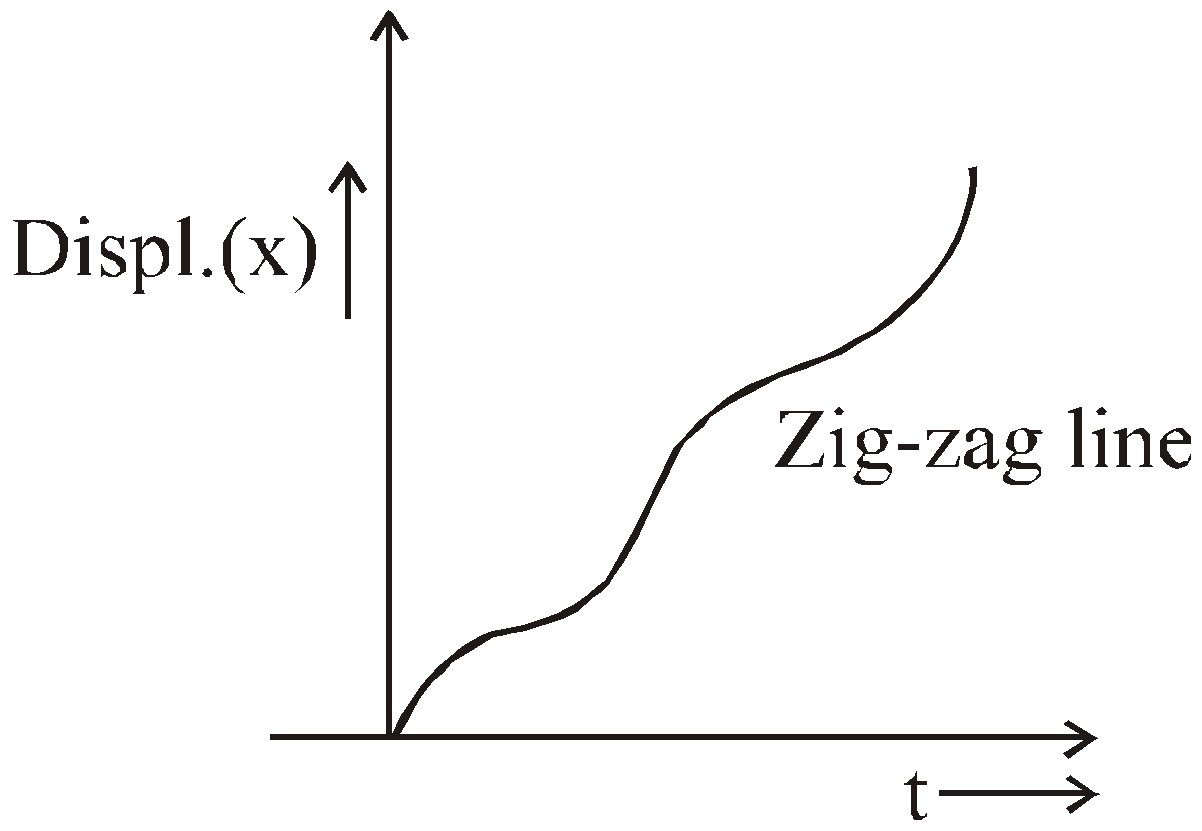• For an accelerated motion the slope of time-displacement curve increases with time while for decelerated motion it decreases with time.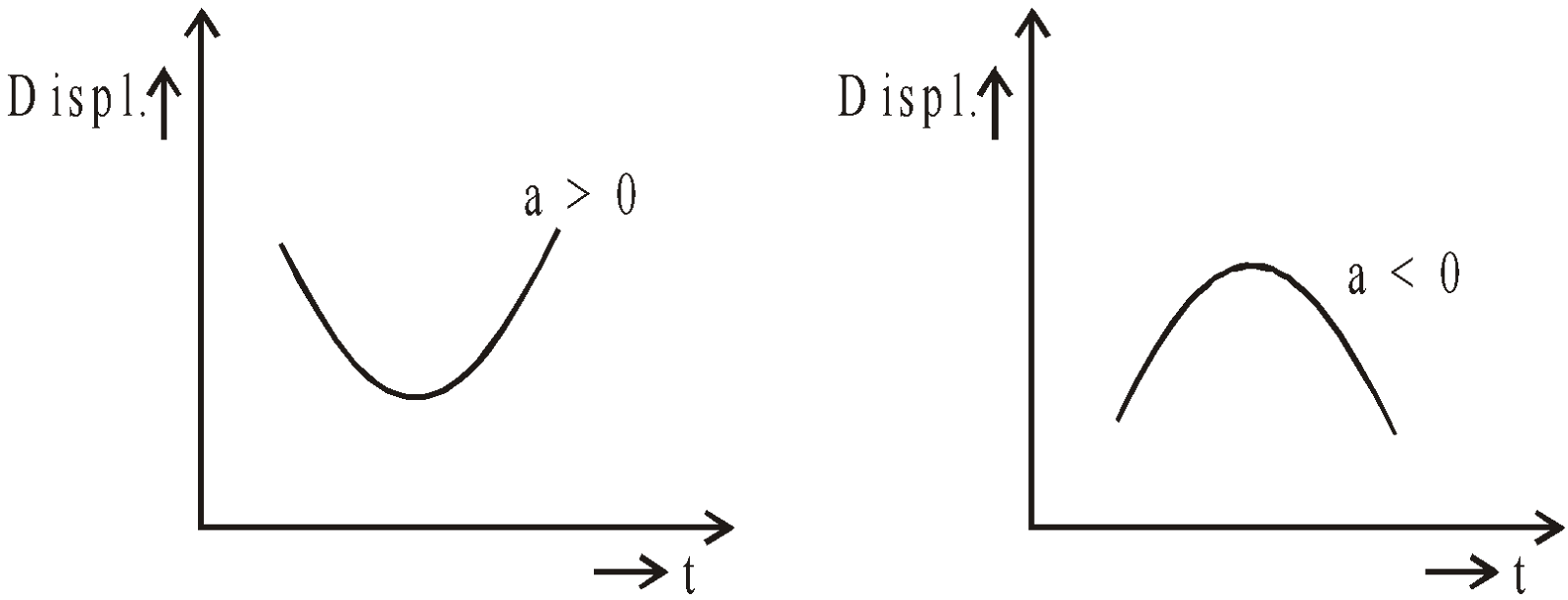• When the particle returns towards the point of reference then the time-displacement line makes an angle θ > 90° with the time axis.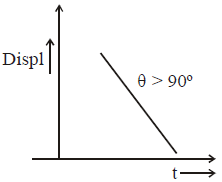#### VELOCITY-TIME GRAPH

In this curve time is plotted along x-axis and velocity is plotted along y-axis.
• When the velocity of the particle is constant or acceleration is zero.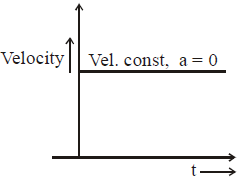• When the particle is moving with a constant acceleration and its initial velocity is zero.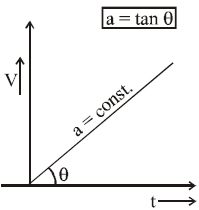• When the particle is moving with constant retardation.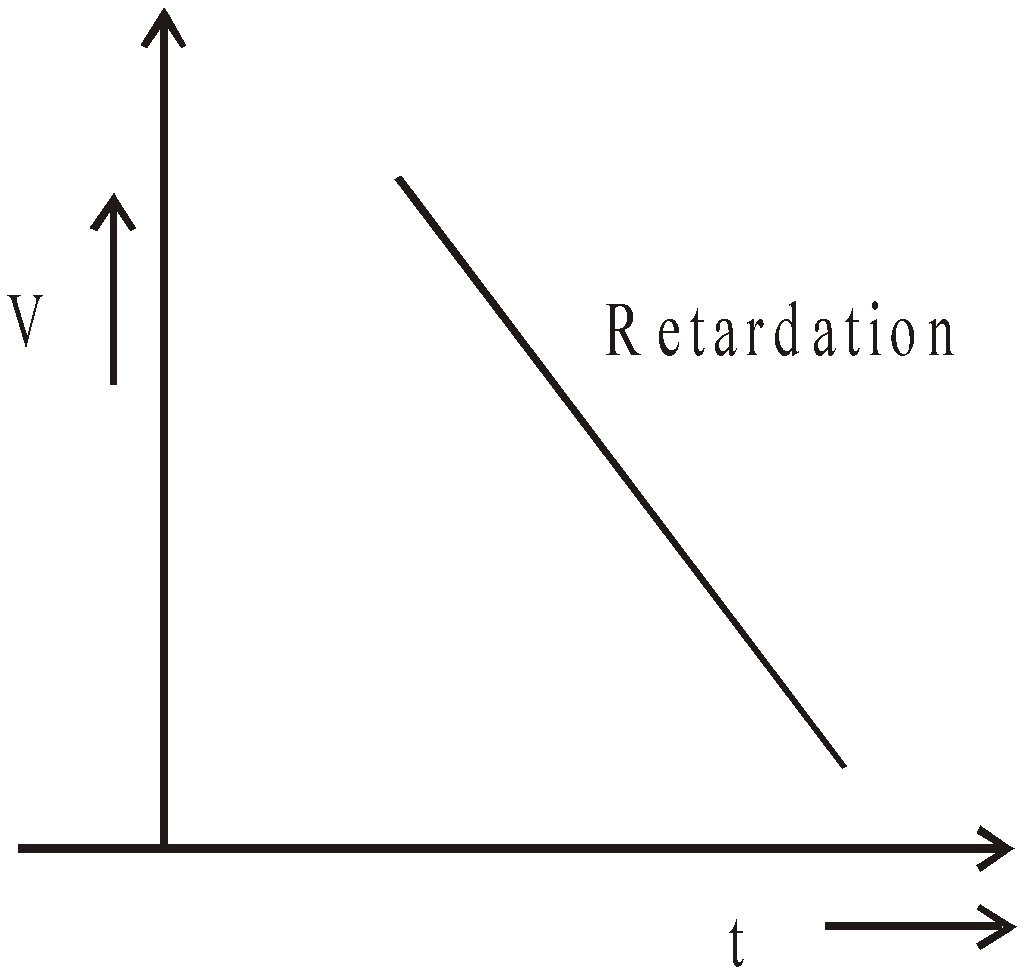• When the particle moves with non-uniform acceleration and its initial velocity is zero.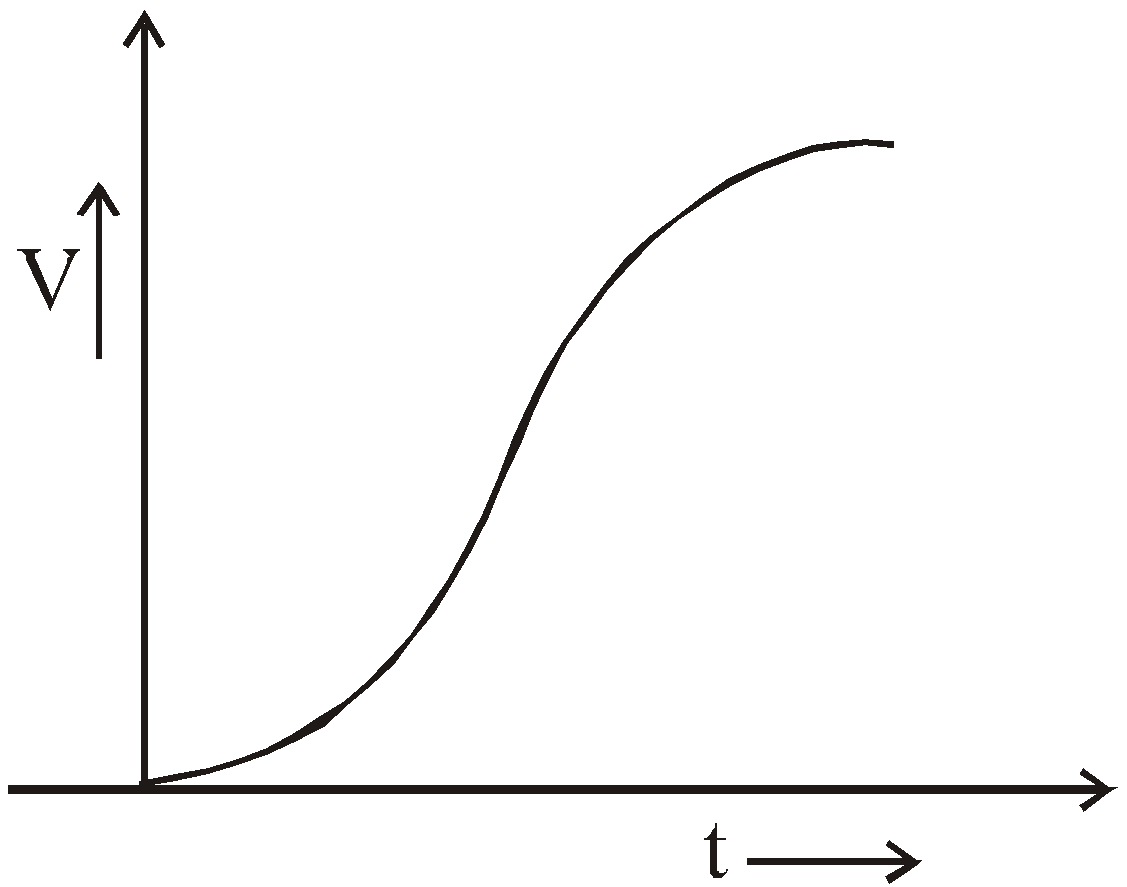• When the acceleration decreases and increases.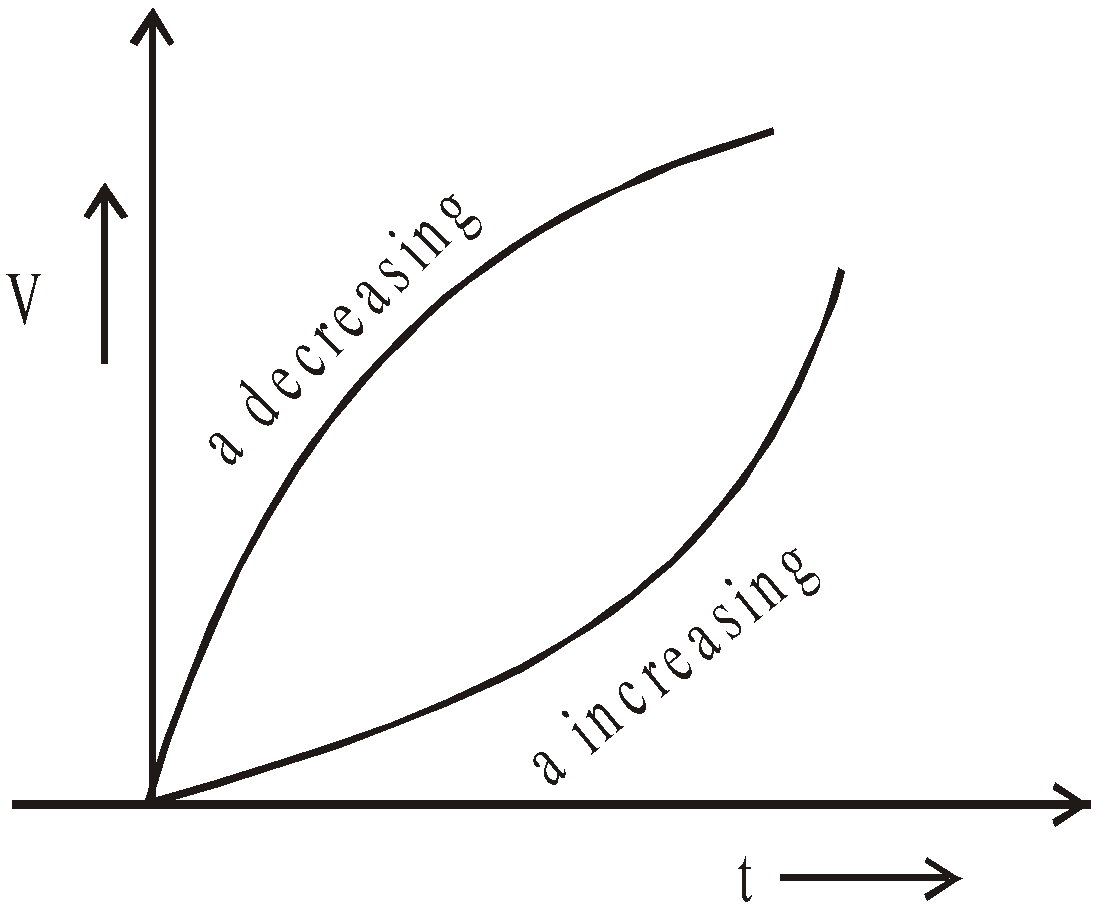• The total area enclosed by the time - velocity curve represents the distance travelled by a body.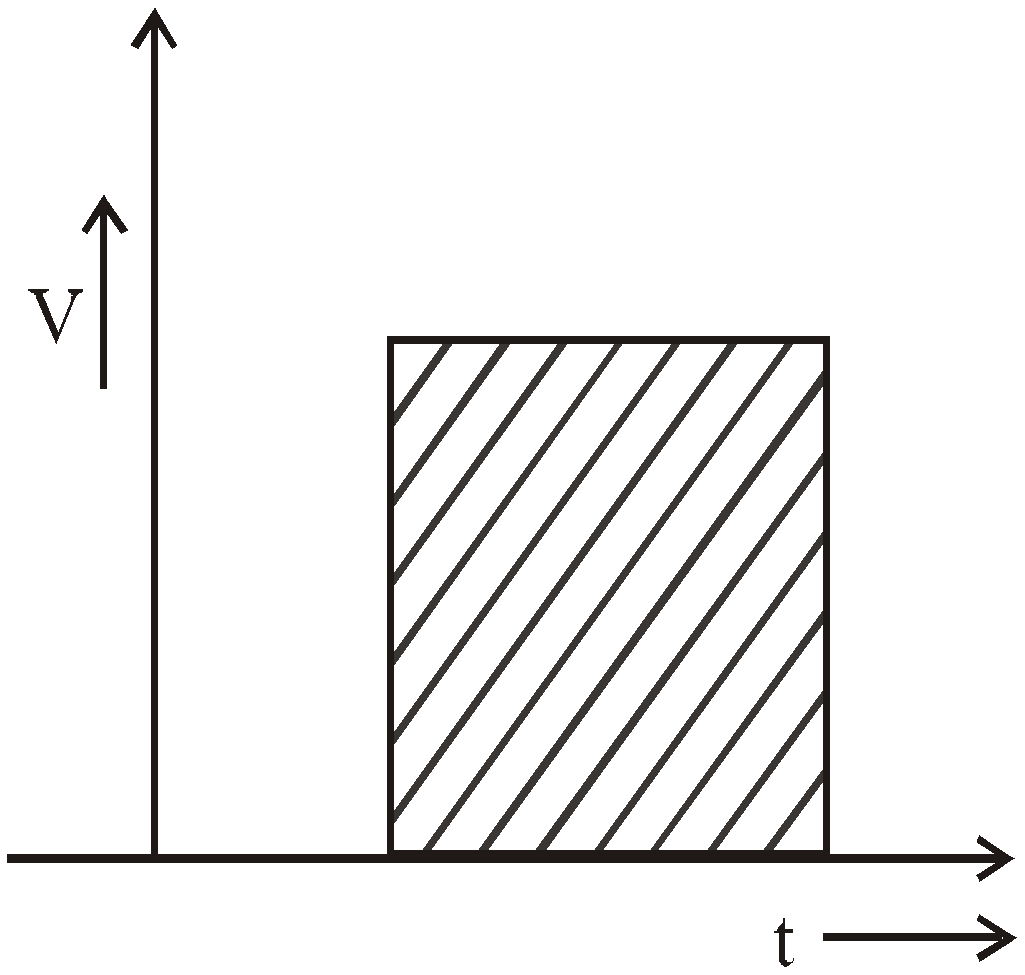While finding displacement through v – t graph, keeping sign under consideration.

#### ACCELERATION-TIME GRAPH

In this curve the time is plotted along X-axis and acceleration is plotted along Y-axis.
• When the acceleration of the particle is zero.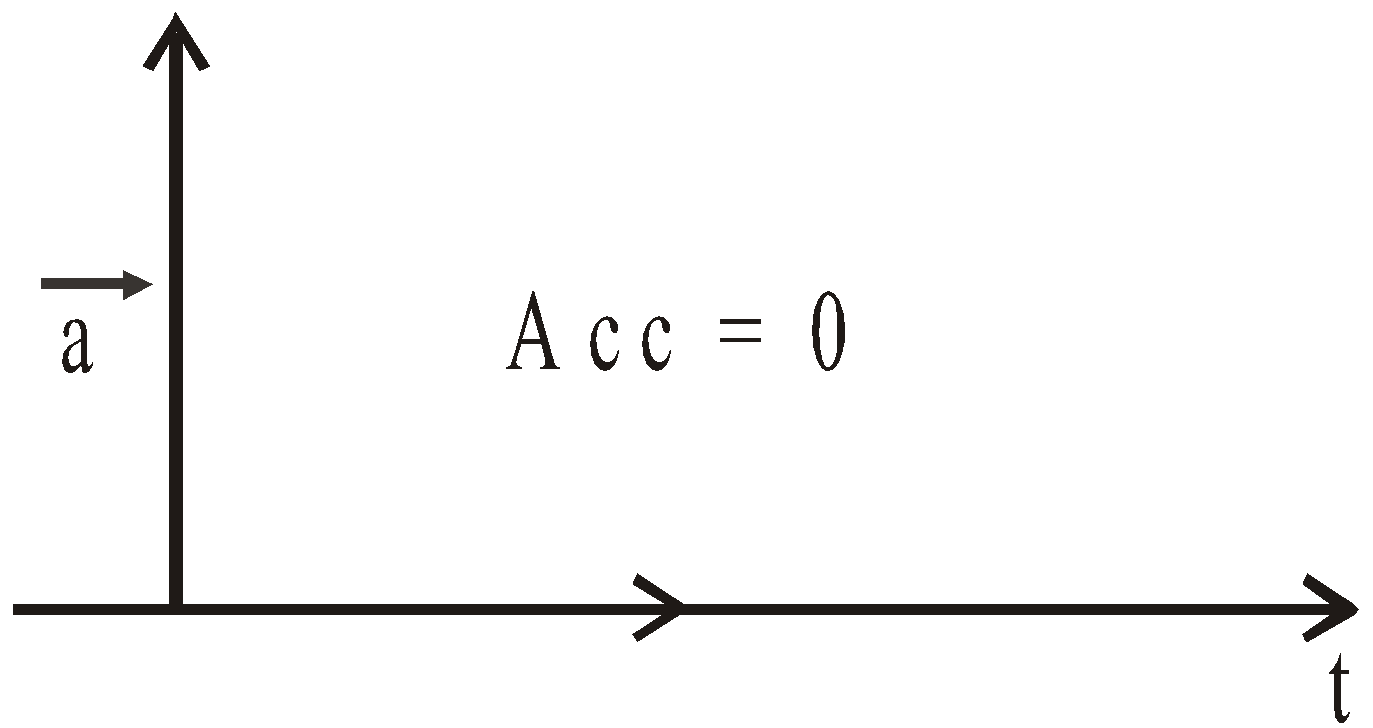• When acceleration is constant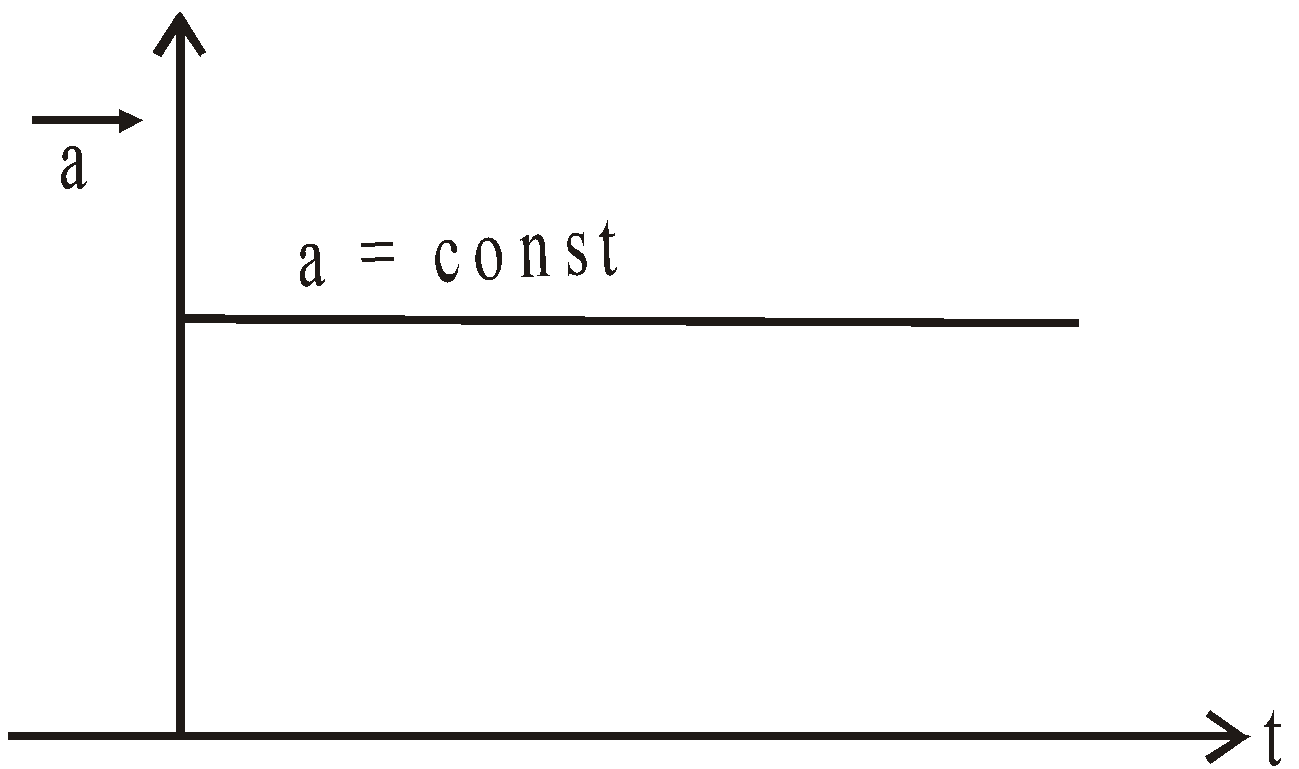• When acceleration is increasing and is positive.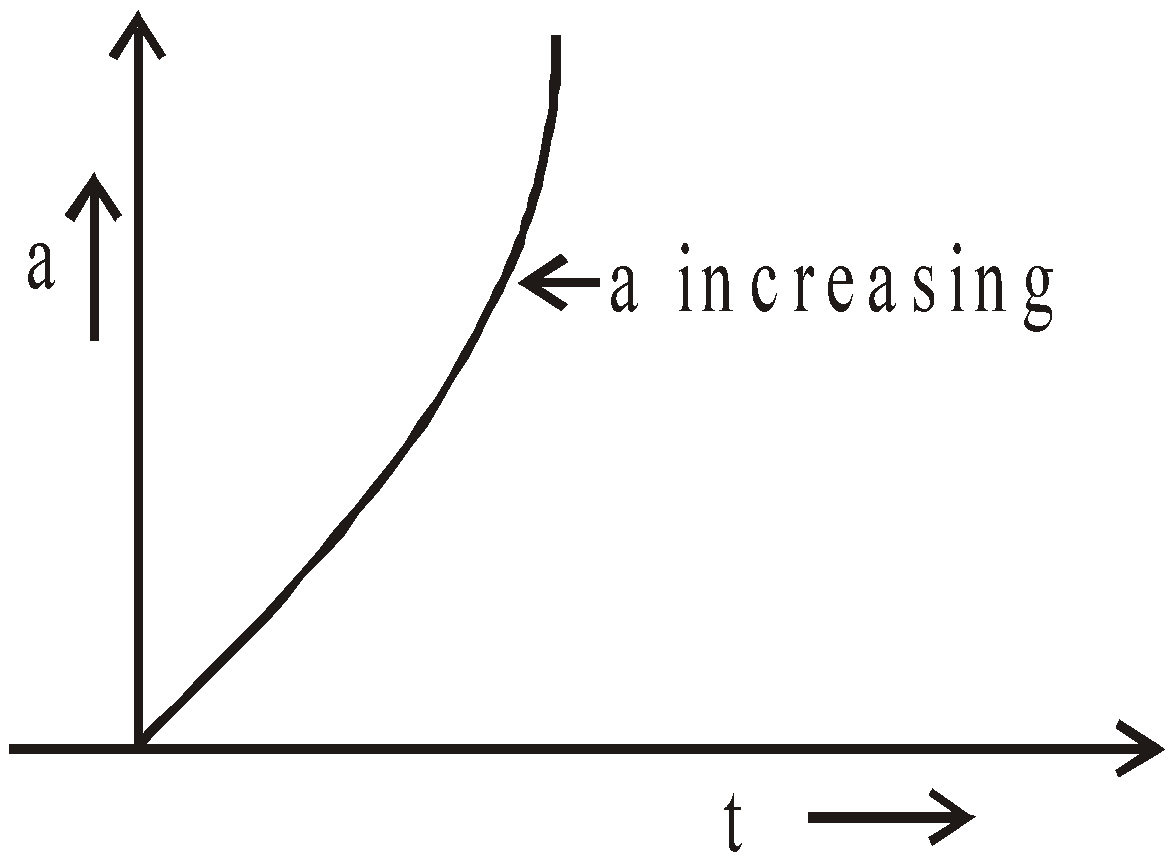• When acceleration is decreasing and is negative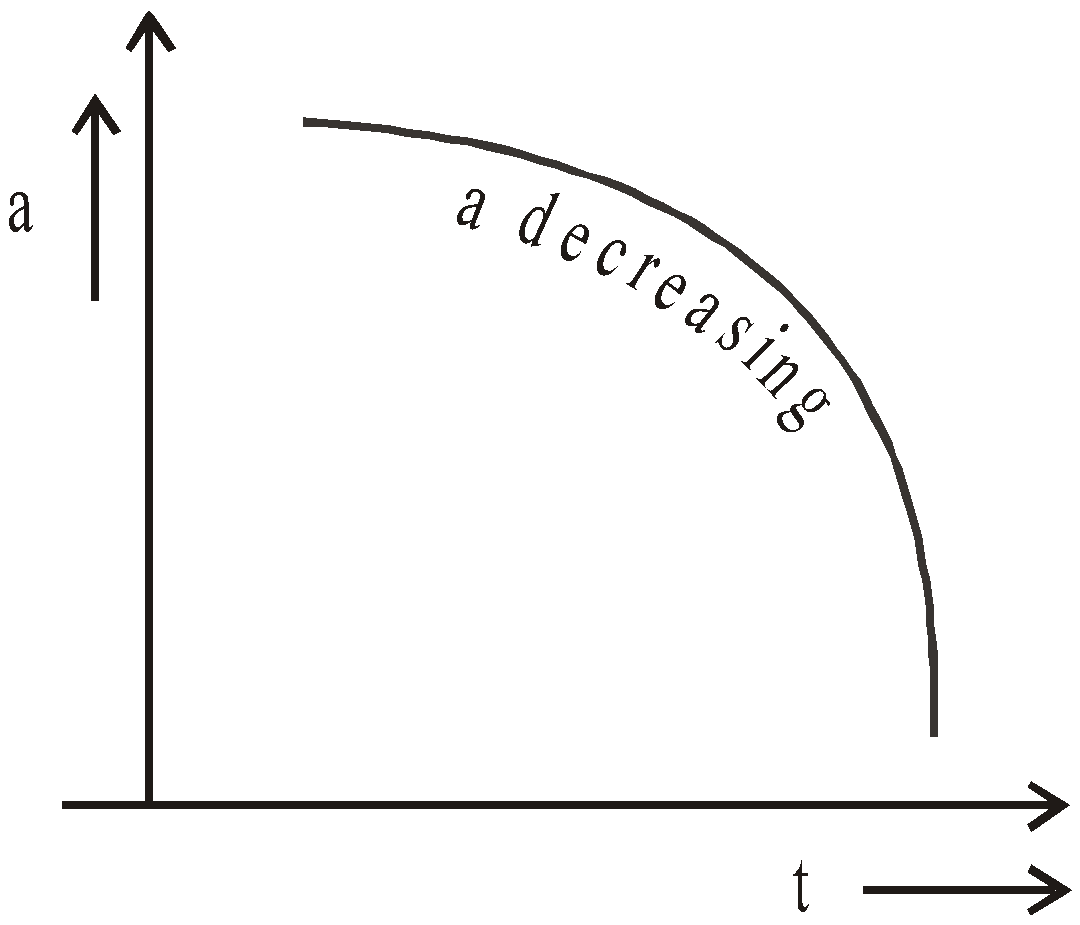• When initial acceleration is zero and rate of change of acceleration is non-uniform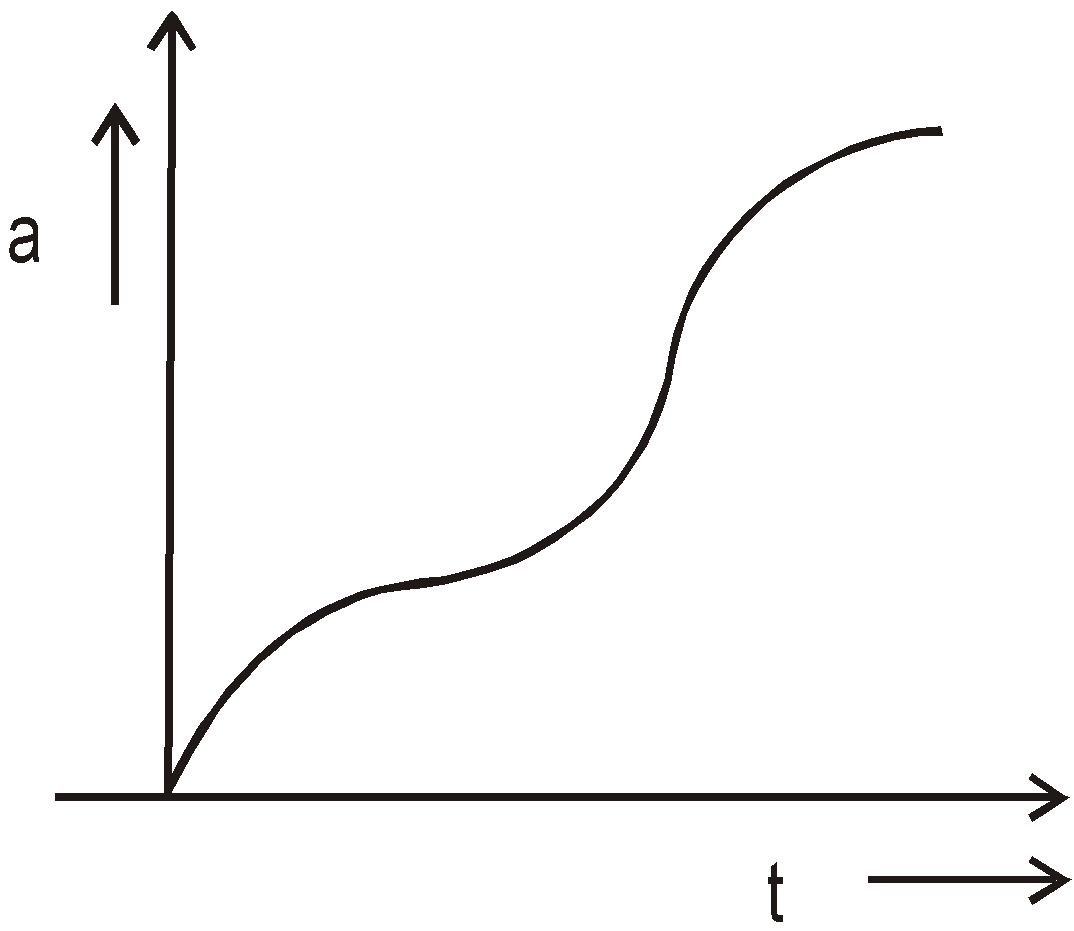• The change in velocity of the particle = area enclosed by the time-acceleration curve.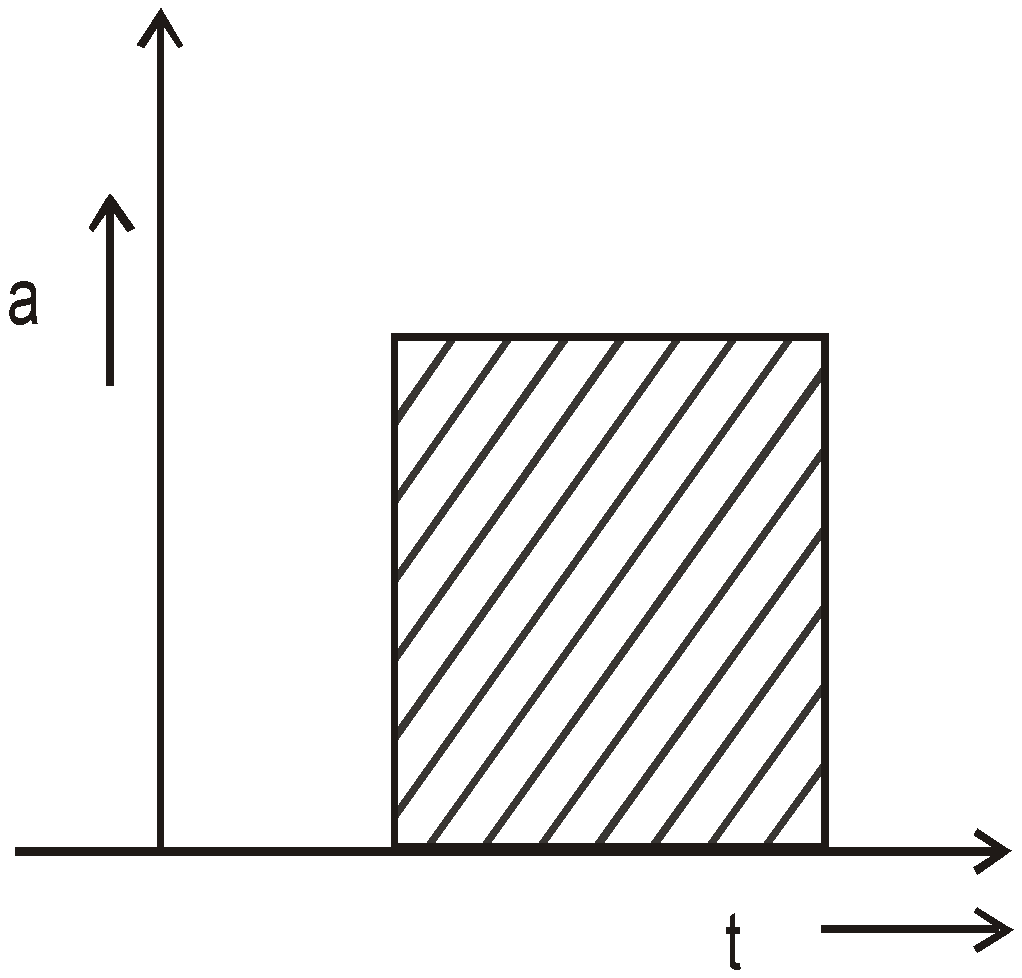### RELATIVE VELOCITY (IN ONE DIMENSION)

The velocity of A relative to B is the velocity with which A appears to be moving w.r.t.an observer who is moving with the velocity of B
Relative velocity of A w.r.t. BSimilarly, relative velocity of B w.r.t. ACase 1 : Bodies moving in same direction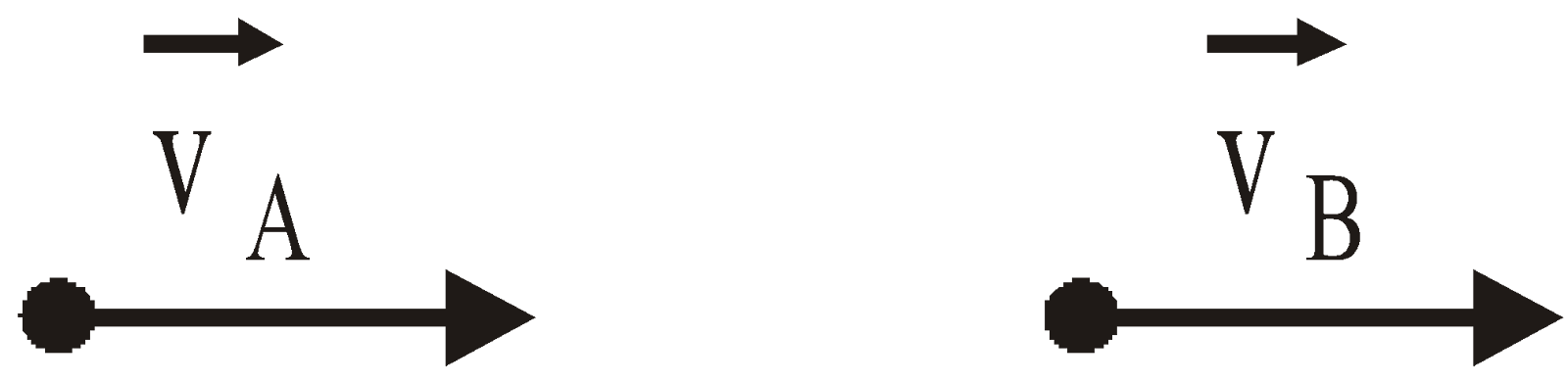⇒  vAB = vA – vB
Case 2 : Bodies moving in opposite direction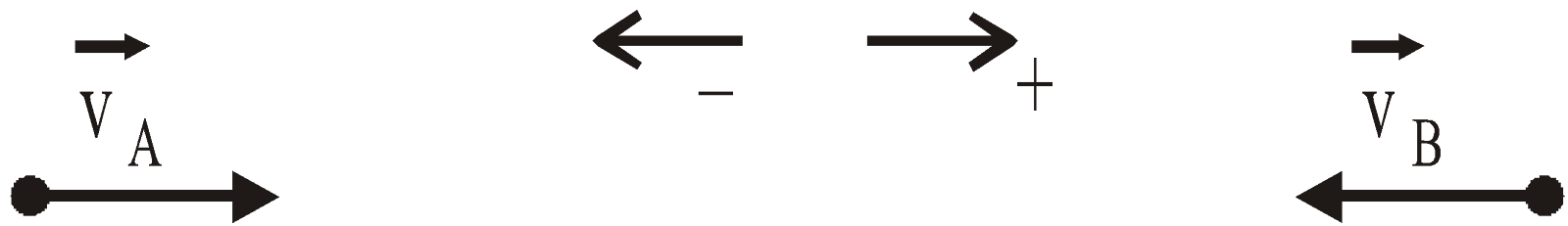⇒  vAB = vA + vB## Want to know more

Please fill in the details below:

## Latest NEET Articles\$type=three\$c=3\$author=hide\$comment=hide\$rm=hide\$date=hide\$snippet=hide

Name

ltr
item
BEST NEET COACHING CENTER | BEST IIT JEE COACHING INSTITUTE | BEST NEET & IIT JEE COACHING: Motion in a Straight Line | Physics Notes for IITJEE/NEET
Motion in a Straight Line | Physics Notes for IITJEE/NEET